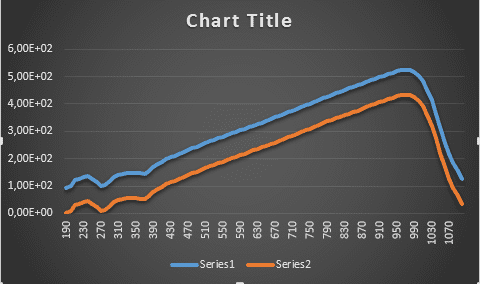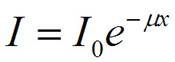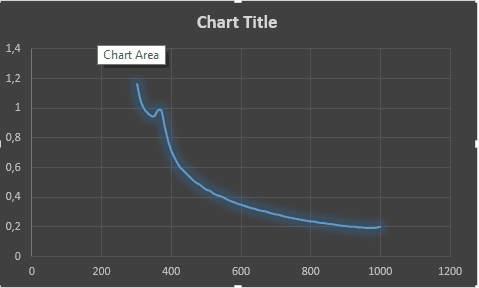# Absorbtion coefficent and radiation curve

## Homework Statement

I have data of an experiment to find absorbtion coefficent of a sample. one curve shows intensity of original beam, the other one is intensity with sample (with wavelenght). Here is the data :## Homework EquationsWhere: I = the intensity of photons transmitted across some distance x
I0 = the initial intensity of photons
s = a proportionality constant that reflects the total probability of a photon being scattered or absorbed
µ = the linear attenuation coefficient
x = distance traveled

## The Attempt at a Solution

I did similar experiment before (not teoricaly). I set the experiment and took original beam intensity(I) and beam with sample intensity(I0). ln (I/I0) gave absorption coefficent.
But now i dont know how to do it with the graph with different wavelengt.

And what is the area under the curve. I couldnt estimate that.
I though the ratio of the areas under curves give the result. Is it correct?

Quantum Defect
Homework Helper
Gold Member

## Homework Statement

I have data of an experiment to find absorbtion coefficent of a sample. one curve shows intensity of original beam, the other one is intensity with sample (with wavelenght). Here is the data :
View attachment 77649

## Homework EquationsWhere: I = the intensity of photons transmitted across some distance x
I0 = the initial intensity of photons
s = a proportionality constant that reflects the total probability of a photon being scattered or absorbed
µ = the linear attenuation coefficient
x = distance traveled

## The Attempt at a Solution

I did similar experiment before (not teoricaly). I set the experiment and took original beam intensity(I) and beam with sample intensity(I0). ln (I/I0) gave absorption coefficent.
But now i dont know how to do it with the graph with different wavelengt.
It looks like you have an Excel spereadsheet with data. You can do the same calculation that you did for a single point with the column vectors in the spreadsheet. Series1 = I0 ; Series 2 = I.

You can rearrange the equation above to get: ln (I/I0) = -mu x if x = path length, how do you calculate mu at each point?

With small intensities, you are likely to get nonsense -- values at short wavelength (<300 nm) will likely be nonsense.

To integrate, you can use Euler's method for numerical integration -- that should be good enough -- but I would limit the integration to where the curve for mu is smooth.

I did -ln(I/I0) and the result is here:It doesnt seem right to me. Absoprtion coefficent doesnt related with wavelenght acourding to lambert beer law. am i right?
So i think it should be like constant not exponential.

Quantum Defect
Homework Helper
Gold Member
I did -ln(I/I0) and the result is here:
View attachment 77656
It doesnt seem right to me. Absoprtion coefficent doesnt related with wavelenght acourding to lambert beer law. am i right?
So i think it should be like constant not exponential.
The absorbance of most things depends upon wavelength. What were you measuring the absorbance of?

In your spectrum, you see larger absorbance at shorter wavelength, this is quite common, as the electronic transitions of many simple molecules & materials are at higher energy, shorter wavelength.

c.f. http://www.chemistrymag.org/cji/2003/056049pe.htm

http://www.chemistrymag.org/cji/2003/images/05604906.gif

Last edited:
It s CdSe.
So wavelength is another variable for absoption.
My main task is to compare this teorotical result with experimental result.
I did the experiment with many dichoric filters. For example with red filter the absorption was 1,09. when i evaluate teoric curve the red area (615-730) the avarage absorption is0,302.
green filter exp: 1,020, teoric 0,4522
blue exp:1,02 teoric 0,5949

first idea was the ratio between them is about distance that light traveled. But as you see the ratio isnt stable. Then you say wavelength is another variable (and i agree with you). now this is my new problem. Do you have any idea. I am stucked